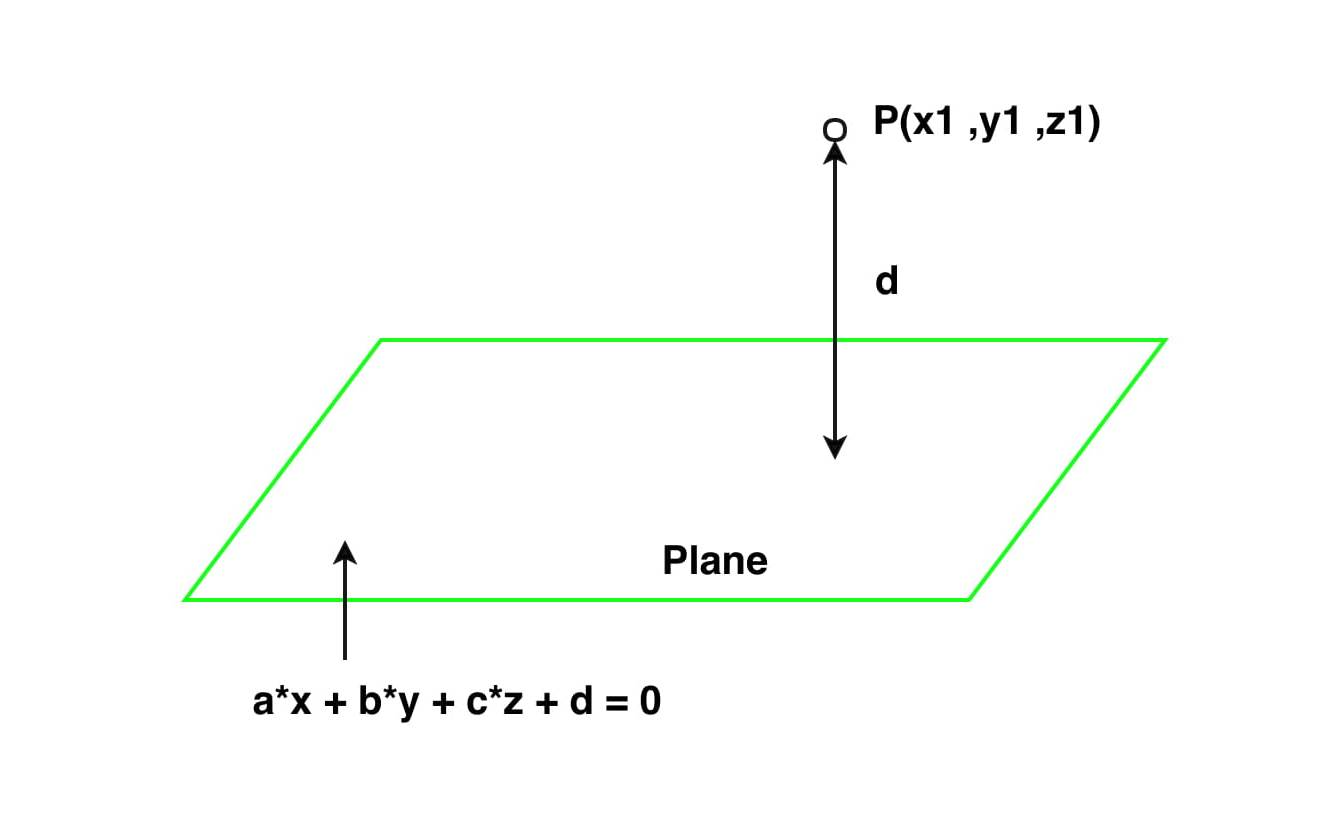# Distance between a point and a Plane in 3 D

• Last Updated : 22 Oct, 2021

You are given a points (x1, y1, z1) and a plane a * x + b * y + c * z + d = 0. The task is to find the perpendicular(shortest) distance between that point and the given Plane.Examples :

Input: x1 = 4, y1 = -4, z1 = 3, a = 2, b = -2, c = 5, d = 8
Output: Perpendicular distance is 6.78902858227
Input: x1 = 2, y1 = 8, z1 = 5, a = 1, b = -2, c = -2, d = -1
Output: Perpendicular distance is 8.33333333333

Approach: The perpendicular distance (i.e shortest distance) from a given point to a Plane is the perpendicular distance from that point to the given plane. Let the co-ordinate of the given point be (x1, y1, z1)
and equation of the plane be given by the equation a * x + b * y + c * z + d = 0, where a, b and c are real constants.
The formula for distance between a point and Plane in 3-D is given by:

`Distance = (| a*x1 + b*y1 + c*z1 + d |) / (sqrt( a*a + b*b + c*c))`

Below is the implementation of the above formulae:

## C++

 `// C++ program to find the``// Perpendicular(shortest)``// distance between a point``// and a Plane in 3 D.``#include``#include` `using` `namespace` `std;` `// Function to find distance``void` `shortest_distance(``float` `x1, ``float` `y1,``                       ``float` `z1, ``float` `a,``                       ``float` `b, ``float` `c,``                       ``float` `d)``{``    ``d = ``fabs``((a * x1 + b * y1 +``              ``c * z1 + d));``    ``float` `e = ``sqrt``(a * a + b *``                   ``b + c * c);``    ``cout << ``"Perpendicular distance is "``         ``<< (d / e);``        ``return``;``}` `// Driver Code``int` `main()``{``    ``float` `x1 = 4;``    ``float` `y1 = -4;``    ``float` `z1 = 3;``    ``float` `a = 2;``    ``float` `b = -2;``    ``float` `c = 5;``    ``float` `d = 8;` `    ``// Function call``    ``shortest_distance(x1, y1, z1,``                      ``a, b, c, d);``}` `// This code is contributed``// by Akanksha Rai(Abby_akku)`

## C

 `// C program to find the Perpendicular(shortest)``// distance between a point and a Plane in 3 D.` `#include``#include` `// Function to find distance``void` `shortest_distance(``float` `x1, ``float` `y1, ``float` `z1,``                    ``float` `a, ``float` `b, ``float` `c, ``float` `d)``{``    ``d = ``fabs``((a * x1 + b * y1 + c * z1 + d));``    ``float` `e = ``sqrt``(a * a + b * b + c * c);``    ``printf``(``"Perpendicular distance is %f"``, d/e);``        ``return``;``}` `// Driver Code``int` `main()``{``    ``float` `x1 = 4;``    ``float` `y1 = -4;``    ``float` `z1 = 3;``    ``float` `a = 2;``    ``float` `b = -2;``    ``float` `c = 5;``    ``float` `d = 8;` `    ``// Function call``    ``shortest_distance(x1, y1, z1, a, b, c, d);``}``// This code is contributed``// by Amber_Saxena.`

## Java

 `// Java program to find the``// Perpendicular(shortest)``// distance between a point``// and a Plane in 3 D.``import` `java .io.*;` `class` `GFG``{``    ` `// Function to find distance``static` `void` `shortest_distance(``float` `x1, ``float` `y1,``                              ``float` `z1, ``float` `a,``                              ``float` `b, ``float` `c,``                              ``float` `d)``{``    ``d = Math.abs((a * x1 + b *``                 ``y1 + c * z1 + d));``    ``float` `e = (``float``)Math.sqrt(a * a + b *``                               ``b + c * c);``    ``System.out.println(``"Perpendicular distance "` `+``                                   ``"is "` `+ d / e);``}` `// Driver code``public` `static` `void` `main(String[] args)``{``    ``float` `x1 = ``4``;``    ``float` `y1 = -``4``;``    ``float` `z1 = ``3``;``    ``float` `a = ``2``;``    ``float` `b = -``2``;``    ``float` `c = ``5``;``    ``float` `d = ``8``;` `    ``// Function call``    ``shortest_distance(x1, y1, z1,``                      ``a, b, c, d);``}``}` `// This code is contributed``// by Amber_Saxena.`

## Python

 `# Python program to find the Perpendicular(shortest)``# distance between a point and a Plane in 3 D.` `import` `math` `# Function to find distance``def` `shortest_distance(x1, y1, z1, a, b, c, d):``    ` `    ``d ``=` `abs``((a ``*` `x1 ``+` `b ``*` `y1 ``+` `c ``*` `z1 ``+` `d))``    ``e ``=` `(math.sqrt(a ``*` `a ``+` `b ``*` `b ``+` `c ``*` `c))``    ``print``(``"Perpendicular distance is"``, d``/``e)``    `  `# Driver Code``x1 ``=` `4``y1 ``=` `-``4``z1 ``=` `3``a ``=` `2``b ``=` `-``2``c ``=` `5``d ``=` `8` `# Function call``shortest_distance(x1, y1, z1, a, b, c, d)     `

## C#

 `// C# program to find the``// Perpendicular(shortest)``// distance between a point``// and a Plane in 3 D.``using` `System;` `class` `GFG``{``    ` `// Function to find distance``static` `void` `shortest_distance(``float` `x1, ``float` `y1,``                              ``float` `z1, ``float` `a,``                              ``float` `b, ``float` `c,``                              ``float` `d)``{``    ``d = Math.Abs((a * x1 + b *``                   ``y1 + c * z1 + d));``    ``float` `e = (``float``)Math.Sqrt(a * a + b *``                               ``b + c * c);``    ``Console.Write(``"Perpendicular distance "` `+``                              ``"is "` `+ d / e);``}` `// Driver code``public` `static` `void` `Main()``{``    ``float` `x1 = 4;``    ``float` `y1 = -4;``    ``float` `z1 = 3;``    ``float` `a = 2;``    ``float` `b = -2;``    ``float` `c = 5;``    ``float` `d = 8;` `    ``// Function call``    ``shortest_distance(x1, y1, z1,``                      ``a, b, c, d);``}``}` `// This code is contributed``// by ChitraNayal`

## PHP

 ``

## Javascript

 ``

Output:

`Perpendicular distance is 6.78902858227`

My Personal Notes arrow_drop_up